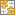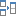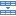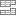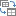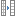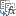# XLOOKUP function in Excel for vertical and horizontal lookup

The tutorial introduces XLOOKUP - the new function for vertical and horizontal lookup in Excel. Left lookup, last match, Vlookup with multiple criteria and a lot more things that used to require a rocket science degree to accomplish have now become as easy as ABC.

Whenever you need to look up in Excel, which function would you use? Is it a cornerstone VLOOKUP or its horizontal sibling HLOOKUP? In a more complex case, will you rely on the canonical INDEX MATCH combination or commit the job to Power Query? The good news is that you do not have choose anymore - all these methods are making way for a more powerful and versatile successor, the XLOOKUP function.

How is XLOOKUP better? In many ways! It can look vertically and horizontally, to the left and above, search with multiple criteria, and even return a whole column or row of data, not just one value. It has taken Microsoft over 3 decades, but finally they've managed to design a robust function that overcomes many frustrating errors and weaknesses of VLOOKUP.

What's the catch? Alas, there is one. Currently XLOOKUP is only available to Microsoft 365 subscribers.

## Excel XLOOKUP function - syntax and uses

The XLOOKUP function in Excel searches a range or an array for a specified value and returns the related value from another column. It can look up both vertically and horizontally and perform an exact match (default), approximate (closest) match, or wildcard (partial) match.

The syntax of the XLOOKUP function is as follows:

XLOOKUP(lookup_value, lookup_array, return_array, [if_not_found], [match_mode], [search_mode])

The first 3 arguments are required and the last three are optional.

• Lookup_value - the value to search for.
• Lookup_array - the range or array where to search.
• Return_array - the range or array from which to return values.
• If_not_found [optional] - the value to return if no match is found. If omitted, an #N/A error is returned.
• Match_mode [optional] - the match type to perform:
• 0 or omitted (default) - exact match. If not found, an #N/A error is returned.
• -1 - exact match or next smaller. If an exact match is not found, the next smaller value is returned.
• 1 - exact match or next larger. If an exact match is not found, the next larger value is returned.
• 2 - wildcard character match.
• Search_mode [optional] - the direction of search:
• 1 or omitted (default) - to search from first to last.
• -1 - to search in reverse order, from last to first.
• 2 - binary search on data sorted ascending.
• -2 - binary search on data sorted descending.

According to Microsoft, binary search is included for advanced users. It is a special algorithm that finds the position of a lookup value within a sorted array by comparing it to the middle element of the array. A binary search is much faster than a regular search but works correctly only on sorted data.

### Basic XLOOKUP formula

To gain more understanding, let's build an Xlookup formula in its simplest form to perform an exact lookup. For this, we will only need the first 3 arguments.

Supposing, you have a summary table with information about the five oceans on the Earth. You want to get the area of a specific ocean input in F1 (lookup_value). With the ocean names in A2:A6 (lookup_array) and areas in C2:C6 (return_array), the formula goes as follows:

`=XLOOKUP(F1, A2:A6, C2:C6)`

Translated into plain English, it says: search for the F1 value in A2:A6 and return a value from C2:C6 in the same row.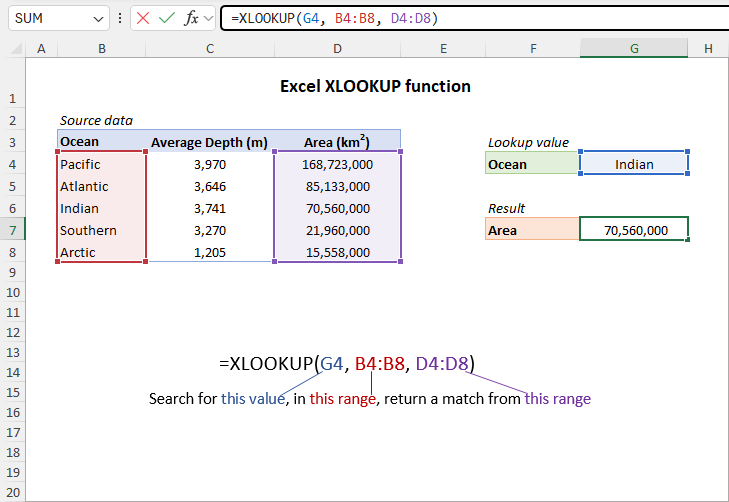No column index numbers, no sorting, no other ridiculous quirks of Vlookup! It just works :)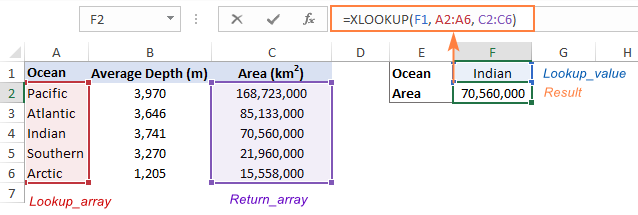## XLOOKUP vs. VLOOKUP

Compared to traditional VLOOKUP, XLOOKUP has many advantages. Here's a list of the best 10 features that blow the doors off any other lookup function in Excel:

1. Vertical and horizontal lookup. The XLOOKUP function got its name due to its ability to look up both vertically and horizontally.
2. Look in any direction: right, left, bottom or up. While VLOOKUP can only search in the leftmost column and HLOOKUP in the topmost row, XLOOKUP has no such limitations. The notorious left lookup in Excel is not a pain anymore!
3. Exact match by default. In most situations, you will be looking for an exact match, and XLOOKUP returns it by default (unlike the VLOOKUP function that defaults to approximate match). Of course, you can get XLOOKUP to perform an approximate match too if needed.
4. Partial match with wildcards. When you know only some part of the lookup value, not all of it, a wildcard match comes in handy.
5. Search in reverse order. Earlier, to get the last occurrence, you had to reverse the order of your source data. Now, you simply set the search_mode argument to -1 to force your Xlookup formula to search from the back and return the last match.
6. Return multiple values. By manipulating with the return_array argument, you can pull an entire row or column of data related to your lookup value.
7. Search with multiple criteria. Excel XLOOKUP handles arrays natively, which makes it possible to perform lookup with multiple criteria.
8. If error functionality. Traditionally, we use the IFNA function to trap #N/A errors. XLOOKUP incorporates this functionality in the if_not_found argument allowing to output your own text if no valid match is found.
9. Column insertions/deletions. One of the most irritating issues with VLOOKUP is that adding or removing columns breaks a formula because the return column is identified by its index number. With XLOOKUP, you supply the return range, not number, meaning you can insert and remove as many columns as you need without breaking anything.
10. Better performance. VLOOKUP could slow down your worksheets because it includes the entire table in calculations, which results in processing far more cells than actually needed. XLOOKUP handles only the lookup and return arrays that it truly depends on.

## How to use XLOOKUP in Excel - formula examples

The following examples demonstrate the most useful XLOOKUP features in action. Additionally, you will discover a couple non-trivial uses that will take your Excel lookup skills to a new level.

### Look up vertically and horizontally

Microsoft Excel used to have two functions for different lookup types, each having its own syntax and usage rules: VLOOKUP to look vertically in a column and HLOOKUP to look horizontally in a row.

The XLOOKUP function can do both with the same syntax. The difference is in what you provide for the lookup and return arrays.

For v-lookup, supply columns:

`=XLOOKUP(E1, A2:A6, B2:B6)`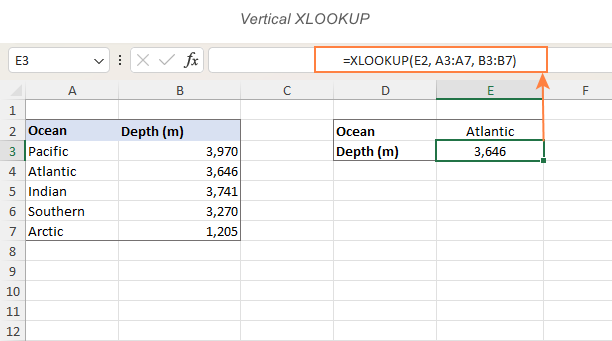For h-lookup, enter rows instead of columns:

`=XLOOKUP(I1, B1:F1, B2:F2)`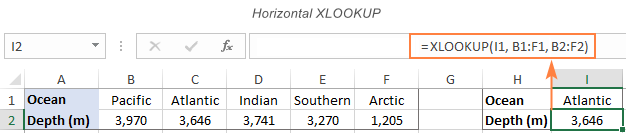### Left lookup performed natively

In earlier versions of Excel, INDEX MATCH formula was the only reliable way to look to the left or above. Now, you no longer need to combine two functions where one would suffice. Just specify the target lookup array, and XLOOKUP will handle it without a problem regardless of its location.

As an example, let's add the Rank column to the left of our sample table. The goal is to get the rank of the ocean input in F1. VLOOKUP would stumble here because it can only return a value from a column to the right of the lookup column. An Xlookup formula copes with ease:

`=XLOOKUP(F1, B2:B6, A2:A6)`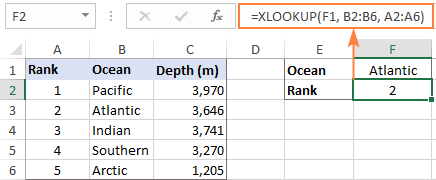In a similar manner, you can look above when searching horizontally in rows.

### XLOOKUP with exact and approximate match

The match behavior is controlled by the 5th argument called match_mode. By default, an exact match is performed.

Please pay attention that even when you choose an approximate match (match_mode set to 1 or -1), the function will still search for an exact match first. The difference is in what it returns if an exact lookup value is not found.

Match_mode argument:

• 0 or omitted - exact match; if not found - #N/A error.
• -1 - exact match; if not found - next smaller item.
• 1 - exact match; if not found - next larger item.

#### Exact match XLOOKUP

This is the option you probably use 99% of the time you do lookup in Excel. Since an exact match is XLOOKUP's default behavior, you can omit match_mode and supply only the first 3 required arguments.

In some situations, however, an exact match won't work. A typical scenario is when your lookup table does not contain all the values, but rather "milestones" or "bounds" like quantity-based discounts, sales-based commissions, etc.

Our sample lookup table shows the correlation between exam scores and grades. As you can see in the screenshot below, an exact match works only when the score of a particular student matches the value in the lookup table exactly (like Christian in row 3). In all other cases, an #N/A error is returned.

`=XLOOKUP(F2, \$B\$2:\$B\$6, \$C\$2:\$C\$6)`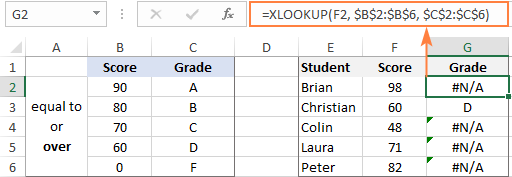To get the grades instead of #N/A errors, we need to look for an approximate match as shown in the next example.

#### Approximate match XLOOKUP

To perform an approximate lookup, set the match_mode argument to either -1 or 1, depending on how your data is organized.

In our case, the lookup table lists the lower bounds of the grades. So, we set match_mode to -1 to search for the next smaller value when an exact match is not found:

`=XLOOKUP(F11, \$B\$11:\$B\$15, \$C\$11:\$C\$15, ,-1)`

For example, Brian has the score of 98 (F2). The formula searches for this lookup value in B2:B6 but cannot find it. Then, it searches for the next smaller item and finds 90, which corresponds to grade A: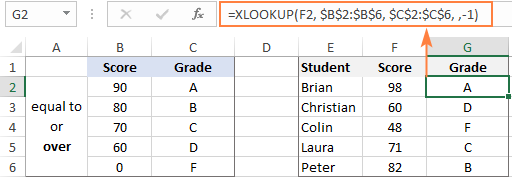If our lookup table contained the upper bounds of the grades, we would set match_mode to 1 to search for the next larger item if an exact match fails:

`=XLOOKUP(F2, \$B\$2:\$B\$6, \$C\$2:\$C\$6, ,1)`

The formula searches for 98 and again cannot find it. This time, it tries to find the next larger value and gets 100, corresponding to grade A: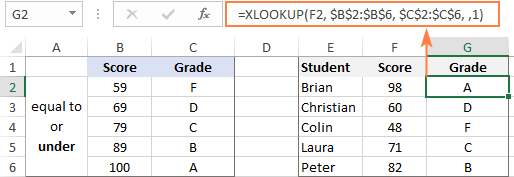Tip. When copying an Xlookup formula to multiple cells, lock the lookup or return ranges with absolute cell references (like \$B\$2:\$B\$6) to prevent them from changing.

### XLOOKUP with partial match (wildcards)

To perform a partial match lookup, set the match_mode argument to 2, which instructs the XLOOKUP function to process the wildcard characters:

• An asterisk (*) - represents any sequence of characters.
• A question mark (?) - represents any single character.

To see how it works, please consider the following example. In column A, you have a few smartphone models and, in column B, their battery capacity. You are curious about the battery of a certain smartphone. The problem is that you are not sure you can type the model name exactly as it appears in column A. To overcome this, enter the part that is definitely there and replace the remaining characters with wildcards.

For example, to get information about the battery of iPhone X, use this formula:

`=XLOOKUP("*iphone X*", A2:A8, B2:B8, ,2)`

Or, input the known part of the lookup value in some cell and concatenate the cell reference with the wildcard characters:

`=XLOOKUP("*"&E1&"*", A2:A8, B2:B8, ,2)`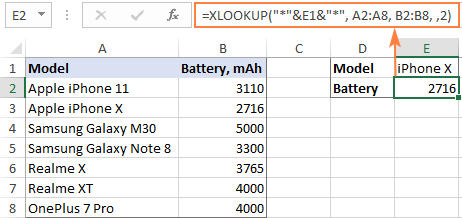### XLOOKUP in reverse order to get last occurrence

In case your table contains several occurrences of the lookup value, you may sometimes need to return the last match. To have it done, configure your Xlookup formula to search in reverse order.

The direction of search is controlled be the 6th argument named search_mode:

• 1 or omitted (default) - searches from first to last value, i.e. top-to-bottom with vertical lookup or left-to-right with horizontal lookup.
• -1 - searches in reverse order from last to first value.

As an example, let's return the last sale made by a specific salesperson. For this, we put together the first three required arguments (G1 for lookup_value, B2:B9 for lookup_array, and D2:D9 for return_array) and put -1 in the 5th argument:

`=XLOOKUP(G1, B2:B9, D2:D9, , ,-1)`

Straightforward and easy, isn't it?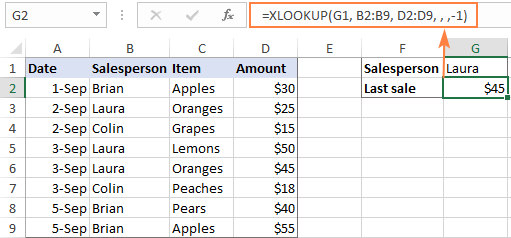### XLOOKUP to return multiple columns or rows

One more amazing feature of XLOOKUP is its ability to return more than one value relating to the same match. All is done with the standard syntax and without any extra manipulations!

From the below table, supposing you want to retrieve all the details pertaining to the salesperson of interest (F2). What you need to do is supply a range, not a singe column or row, for the return_array argument:

`=XLOOKUP(F2, A2:A7, B2:D7)`

You enter the formula in the top-left cell of the results range, and Excel automatically spills the results into adjacent blank cells. In our case, the return array (B2:D7) includes 3 columns (Date, Item and Amount), and all three values are returned into the range G2:I2.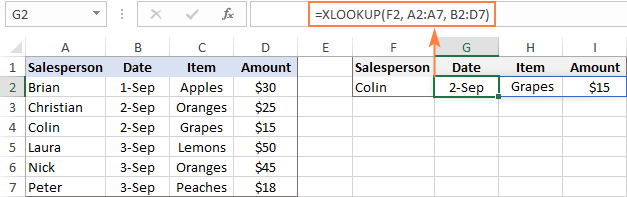If you'd rather arrange the results vertically in a column, nest XLOOKUP into the TRANSPOSE function to flip the returned array:

`=TRANSPOSE(XLOOKUP(G1, A2:A7, B2:D7))`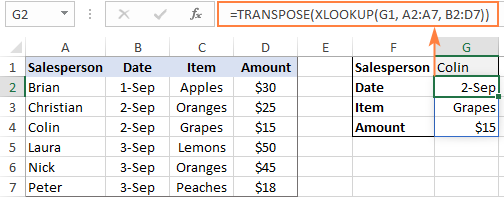In a similar fashion, you can return an entire column of data, say the Amount column. For this, use cell F1 that contains "Amount" as lookup_value, the range A1:D1 containing the column headers as lookup_array and the range A2:D7 containing all data as return_array.

`=XLOOKUP(F1, A1:D1, A2:D7)`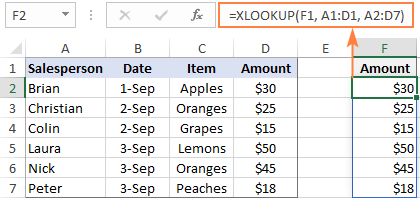Note. Because multiple values are populated into neighboring cells, make sure you have enough blank cells to the right or down. If Excel cannot find enough empty cells, a #SPILL! error occurs.

### XLOOKUP with multiple criteria

Another big advantage of XLOOKUP is that it handles arrays natively. Due to this ability, you can evaluate multiple criteria directly in the lookup_array argument:

XLOOKUP(1, (criteria_range1=criteria1) * (criteria_range2=criteria2) * (…), return_array)

How this formula works: The result of each criteria test is an array of TRUE and FALSE values. The multiplication of the arrays converts TRUE and FALSE into 1 and 0, respectively, and produces the final lookup array. As you know, multiplying by 0 always gives zero, so in the lookup array, only the items that meet all the criteria are represented by 1. And because our lookup value is "1", Excel takes the first "1" in lookup_array (first match) and returns the value from return_array in the same position.

To see the formula in action, let's pull an amount from D2:D10 (return_array) with the following conditions:

• Criteria1 (date) = G1
• Criteria2 (salesperson) = G2
• Criteria3 (item) = G3

With dates in A2:A10 (criteria_range1), salesperson names in B2:B10 (criteria_range2) and items in C2:C10 (criteria_range3), the formula takes this shape:

`=XLOOKUP(1, (B2:B10=G1) * (A2:A10=G2) * (C2:C10=G3), D2:D10)`

Though the Excel XLOOKUP function processes arrays, it works as a regular formula and is completed with a usual Enter keystroke.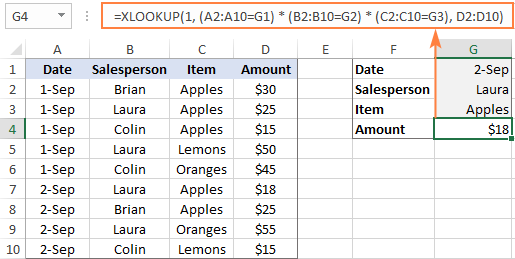The XLOOKUP formula with multiple criteria is not limited to "equal to" conditions. You are free to use other logical operators as well. For example, to filter orders made on the date in G1 or earlier, put "<=G1" in the first criterion:

`=XLOOKUP(1, (A2:A10<=G1) * (B2:B10=G2) * (C2:C10=G3), D2:D10)`

### Two-dimensional XLOOKUP

To find a value at the intersection of a certain row and column, perform the so-called double lookup or matrix lookup. Yep, Excel XLOOKUP can do that too! You simply nest one function inside another:

XLOOKUP(lookup_value1, lookup_array1, XLOOKUP(lookup_value2, lookup_array2, data_values))

How this formula works: The formula is based on XLOOKUP's ability to return an entire row or column. The inner function searches for its lookup value and returns a column or row of related data. That array goes to the outer function as the return_array.

For this example, we are going to find the sales made by a particular salesperson within a certain quarter. For this, we enter the lookup values in H1 (salesperson name) and H2 (quarter), and do a two-way Xlookup with the following formula:

`=XLOOKUP(H1, A2:A6, XLOOKUP(H2, B1:E1, B2:E6))`

Or the other way round:

`=XLOOKUP(H2, B1:E1, XLOOKUP(H1, A2:A6, B2:E6))`

Where A2:A6 are the salesperson names, B1:E1 are quarters (column headers), and B2:E6 are data values.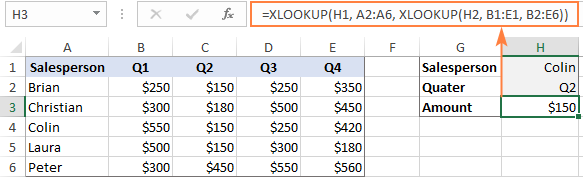A two-way lookup can also be performed with an INDEX Match formula and in a few other ways. For more information, please see Two-way lookup in Excel.

### If Error XLOOKUP

When the lookup value is not found, Excel XLOOKUP returns an #N/A error. Quite familiar and understandable to expert users, it might be rather confusing for novices. To replace the standard error notation with a user-friendly message, type your own text into the 4th argument named if_not_found.

Back to the very first example discussed in this tutorial. If someone inputs an invalid ocean name in E1, the following formula will explicitly tell them that "No match is found":

`=XLOOKUP(E1, A2:A6, B2:B6, "No match is found")`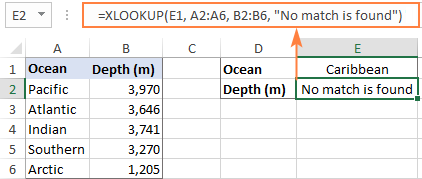Notes:

• The if_not_found argument traps only #N/A errors, not all errors.
• #N/A errors can also be handled with IFNA and VLOOKUP, but the syntax is a bit more complex and a formula is lengthier.

## Excel XLOOKUP not working

If your formula does not work right or results in error, most likely it's because of the following reasons:

### XLOOKUP is not available in my Excel

The XLOOKUP function is not backward compatible. It's only available with Office 365 subscriptions and won't appear in earlier Excel versions.

### XLOOKUP returns wrong result

If your obviously correct Xlookup formula returns a wrong value, chances are that the lookup or return range "shifted" when the formula was copied down or across. To prevent this from happening, be sure to always lock both ranges with absolute cell references (like \$A\$2:\$A\$10).

### XLOOKUP returns #N/A error

An #N/A error just means the lookup value is not found. To fix this, try searching for approximate match or inform your users that no match is found.

### XLOOKUP returns #VALUE error

A #VALUE! error occurs if the lookup and return arrays have incompatible dimensions. For instance, it is not possible to search in a horizontal array and return values from a vertical array.

### XLOOKUP returns #REF error

A #REF! error is thrown when looking up between two different workbooks, one of which is closed. To fix the error, simply open both files.

As you have just seen, XLOOKUP has many awesome features that make it THE function for almost any lookup in Excel. I thank you for reading and hope to see you on our blog next week!

Excel XLOOKUP formula examples (.xlsx file)

### 12 responses to "XLOOKUP function in Excel for vertical and horizontal lookup"

1.Stephane says:

Hi
I am trying to work out this formula =IF(A2>0,"Non Compliance","Compliance")but i want to exclude blank cells. How do i do that?

thanks
Stephane

•Svetlana Cheusheva (Ablebits.com Team) says:

Hi Stephane,
You can nest another IF function that checks for blanks, like this:
=IF(A2="","", IF(A2>0,"Non Compliance","Compliance"))

2.Hamada says:

I couldn't find xlookup in excel 2019

•Svetlana Cheusheva (Ablebits.com Team) says:

Currently XLOOKUP is only available to users of the Office 365 Insiders channel.

•Svetlana Cheusheva (Ablebits.com Team) says:

Update: the XLOOKUP function is now available in the Monthly channel of Office 365 subscriptions. Starting in July 2020, it is planned to be included in the Semi-Annual channel.

3.Robert Kellogg says:

Is it possible to utilize an XLOOKUP in conjunction with a range? I'd like to return a value based on a range that varies depending on the lookup criteria.

Example. Criteria one is a customer name, Criteria two is their sales, Result/Return is a order frequency. There is 5 possible return values of what the order frequency should be based on the range of sales and the customer name. There are different ranges based on customer names.

•Alexander Trifuntov (Ablebits.com Team) says:

Hello Robert!
Read carefully the section above "XLOOKUP with multiple criteria" You did not explain what you mean by "order frequency".
I’m sorry but your task is not entirely clear to me. For me to be able to help you better, please describe your task in more detail. Please specify what you were trying to find, what formula you used and what problem or error occurred. Give an example of the source data and the expected result.
It’ll help me understand it better and find a solution for you. Thank you.

4.JoJo says:

I have multiple Xlookup's running in my workbook, but I'm having issues with a particular one. I have a column with pay type data (regular time, overtime) and another column where the job name is listed. I am attempting to use xlookup to determine which pay type AND if the job name is one of 3 possibilities (referencing a range of cells for that data) have it return a job type number listed in another range of cells. My current formula is =XLOOKUP(\$E2,AC2:AC5&(\$T1,AE2:AE5),AD2:AD5,E2)which returns "regular time" as the answer (which I only want it to do if it won't be replacing the data with information from the proper range). I have to be missing part of the query, but I'm not sure where.

•Alexander Trifuntov (Ablebits.com Team) says:

Hello!
I’m sorry but your task is not entirely clear to me. Could you please describe it in more detail?
What do you want to determine using this formula? Pay type? Job type? What is written in cell E2? What is written in cell T1? You are using 3 ranges of AC2: AC5 AE2: AE5 AD2: AD5. What is written in them? Without this, I cannot understand the logic of your formula

5.Olivier says:

Hi Svetlana,
Very complete but I am stuck with a more complex issue.
I need to get a value based on 3 criteria, including one that is an approximate (i.e. lookup value would be within a range)
My formula looks like this:
=xlookup(Value01&Value02&Value03,Range01&Range02&Range03,ReturnRange,,-1) where value 03 is the approximate crlteria.

The ranges are same lenghts and side by side. It should work however it gives me random return values, never consistent.

6.Dave Quinn says:

Thanks this is great! For some reason the search in reverse order is not working.
Using your example my sheet has (=XLOOKUP(G1,B2:B9,D2:D9, ,-1)) still gives me \$25 as the result, not \$45.
All the other examples seem to work great

•Svetlana Cheusheva (Ablebits.com Team) says:

Hi Dave,

Thank you for pointing out this issue!

It looks like Microsoft has changed the order of arguments in the XLOOKUP function! At the moment of writing, this function was a beta available for Office Insiders. That time, search_mode that controls the direction of search was the 5th argument. Now, it's the last (6th) argument. I will review and update all the affected examples ASAP.

In the meantime, please use this formula to get the last occurrence:
=XLOOKUP(G1, B2:B9, D2:D9, , ,-1)

Ultimate Suite for Microsoft Excel